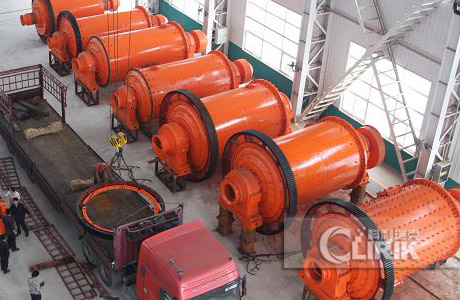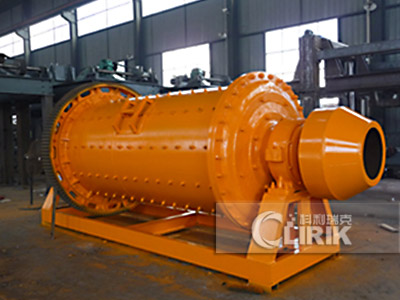# 2.4 m Ball Mill speed — model

Posted on Posted in news

Ball mill is a kind of grinding equipment with steel ball as grinding medium. For different processing capacity, there are many specifications of ball mills to choose from. The 2.4-meter ball mill refers to a ball mill with a diameter of 2.4 meters. Its processing capacity is 7.2 ~ 110t / h. It belongs to a medium-sized mill and can meet the needs of small and medium-sized mills. Many friends are more concerned about the speed of the ball mill. Here Clirik briefly introduces you how much the speed of the 2.4 meter ball mill and what are the models of the 2.4 meter ball mill. I hope to provide some reference when you choose the equipment.#### Speed ​​of 2.4 m Ball Mill

There are two concepts of the speed of the ball mill, one is the critical speed, and the other is the working speed. The so-called critical speed is that when the barrel of the ball mill rotates, the steel balls in the barrel will not fall or fall, but will follow the barrel. Rotating together, the speed that can maintain this state becomes the optimal speed of the ball mill. The formula for calculating the critical speed is: n = 424 / √D, where n is the critical speed of the ball mill (r / min), and D is the inner diameter of the cylinder (cm).

What about the working speed? In fact, after repeated experiments and practice, it has been basically determined that the working speed of the ball mill is 76% of the critical speed. According to the above formula, we can calculate: a ball mill with a diameter of 2.4 meters, assuming a cylinder The thickness of the inner liner is 5cm, then the inner diameter D = 235cm. According to the calculation, the critical speed is n = 424 / √235 = 27.66r / min, and the working speed is nX76% = 21.02r / min.#### Model of 2.4 m Ball Mill

Above we introduced the speed of the 2.4 m ball mill, so do you know what specifications and models of the 2.4 m ball mill? What are their parameters? Here are some of the 2.4 m ball mills produced by Shanghai Clirik for you to choose. The ball mills produced by Clirik include four types: lattice type, overflow type, energy-saving lattice type and energy-saving overflow type. Each of them contains a 2.4-meter ball mill. The table below lists the detailed technical parameters of each model :

 Model Rotary speed（r/min) weight(t) Input size(mm) Discharge size(mm) Capacity(t/h) Motor power (kw) Weight (t) Ф2400×4500 21 30 ≤25 0.074-0.4 18-45 320 65 Ф2400×8000 20 36 ≤25 0.074-0.4 20-48 410 81

The above is the introduction of the speed and model of the 2.4-meter ball mill. In the selection of ball mills, we have to consider a variety of factors, including processing capacity, grain size of incoming and outgoing mills, the nature and hardness of the material, whether it is open or closed, and subsequent selection processes. . Only in this way can you choose the right equipment. If you have a selection request, please contact Clirik through the contact information below.